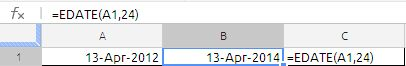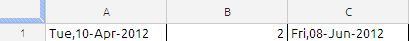# EDATE

The EDATE function returns a date that is “X” number of months before or after a given date.

EDATE(start_date, months)

start-date:  The starting date. start-date is a date value.

months: The number of months before or after the starting date. months is a number value. A negative months is used to specify a number of months before the starting date and a positive months is used to specify a number of months after the starting date.

Example 1:

Suppose we have to issue bank guarantee for a period of 24 month from current date of 13-APR-2012

to calculate the validity period we use the EDATE function as shown in the image below:Example 2:

the bill due date in 2 months from the current date which is 10-APR-2012 . if the due date happens to be saturday and sunday then the due date will be last Friday.

We can solve this using the Weekday function and edate function. the formulas are clearly explained in the below image.the formula in cell C1 is

=EDATE(A1,B1)-IF(WEEKDAY(EDATE(A1,B1),2)>5,WEEKDAY(EDATE(A1,B1),2)-5,0)

The =WEEKDAY() function has been used to identify the actual weekday number of the end date. If the weekday number is 6 or 7, (Sat or Sun), then 5 is subtracted from the =EDATE() to ensure the end of contract falls on a Friday.